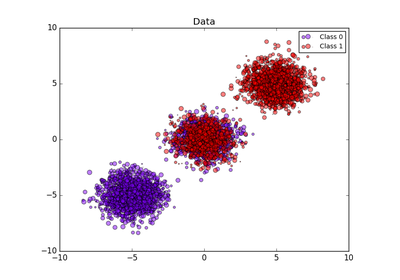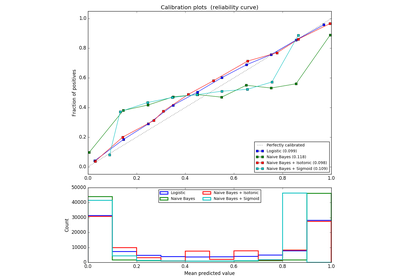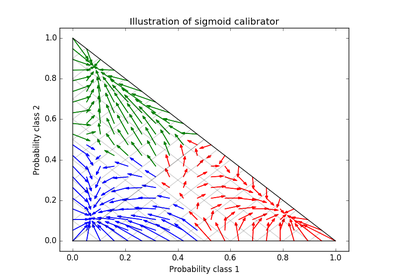# `sklearn.calibration`.CalibratedClassifierCV¶

class `sklearn.calibration.``CalibratedClassifierCV`(base_estimator=None, method='sigmoid', cv=3)[source]

Probability calibration with isotonic regression or sigmoid.

With this class, the base_estimator is fit on the train set of the cross-validation generator and the test set is used for calibration. The probabilities for each of the folds are then averaged for prediction. In case that cv=”prefit” is passed to __init__, it is assumed that base_estimator has been fitted already and all data is used for calibration. Note that data for fitting the classifier and for calibrating it must be disjoint.

Read more in the User Guide.

Parameters: base_estimator : instance BaseEstimator The classifier whose output decision function needs to be calibrated to offer more accurate predict_proba outputs. If cv=prefit, the classifier must have been fit already on data. method : ‘sigmoid’ or ‘isotonic’ The method to use for calibration. Can be ‘sigmoid’ which corresponds to Platt’s method or ‘isotonic’ which is a non-parametric approach. It is not advised to use isotonic calibration with too few calibration samples `(<<1000)` since it tends to overfit. Use sigmoids (Platt’s calibration) in this case. cv : integer, cross-validation generator, iterable or “prefit”, optional Determines the cross-validation splitting strategy. Possible inputs for cv are: None, to use the default 3-fold cross-validation, integer, to specify the number of folds. An object to be used as a cross-validation generator. An iterable yielding train/test splits. For integer/None inputs, if `y` is binary or multiclass, `sklearn.model_selection.StratifiedKFold` is used. If `y` is neither binary nor multiclass, `sklearn.model_selection.KFold` is used. Refer User Guide for the various cross-validation strategies that can be used here. If “prefit” is passed, it is assumed that base_estimator has been fitted already and all data is used for calibration. classes_ : array, shape (n_classes) The class labels. calibrated_classifiers_: list (len() equal to cv or 1 if cv == “prefit”) : The list of calibrated classifiers, one for each crossvalidation fold, which has been fitted on all but the validation fold and calibrated on the validation fold.

References

 [R128] Obtaining calibrated probability estimates from decision trees and naive Bayesian classifiers, B. Zadrozny & C. Elkan, ICML 2001
 [R129] Transforming Classifier Scores into Accurate Multiclass Probability Estimates, B. Zadrozny & C. Elkan, (KDD 2002)
 [R130] Probabilistic Outputs for Support Vector Machines and Comparisons to Regularized Likelihood Methods, J. Platt, (1999)
 [R131] Predicting Good Probabilities with Supervised Learning, A. Niculescu-Mizil & R. Caruana, ICML 2005

Methods

 `fit`(X, y[, sample_weight]) Fit the calibrated model `get_params`([deep]) Get parameters for this estimator. `predict`(X) Predict the target of new samples. `predict_proba`(X) Posterior probabilities of classification `score`(X, y[, sample_weight]) Returns the mean accuracy on the given test data and labels. `set_params`(\*\*params) Set the parameters of this estimator.
`__init__`(base_estimator=None, method='sigmoid', cv=3)[source]
`fit`(X, y, sample_weight=None)[source]

Fit the calibrated model

Parameters: X : array-like, shape (n_samples, n_features) Training data. y : array-like, shape (n_samples,) Target values. sample_weight : array-like, shape = [n_samples] or None Sample weights. If None, then samples are equally weighted. self : object Returns an instance of self.
`get_params`(deep=True)[source]

Get parameters for this estimator.

Parameters: deep : boolean, optional If True, will return the parameters for this estimator and contained subobjects that are estimators. params : mapping of string to any Parameter names mapped to their values.
`predict`(X)[source]

Predict the target of new samples. Can be different from the prediction of the uncalibrated classifier.

Parameters: X : array-like, shape (n_samples, n_features) The samples. C : array, shape (n_samples,) The predicted class.
`predict_proba`(X)[source]

Posterior probabilities of classification

This function returns posterior probabilities of classification according to each class on an array of test vectors X.

Parameters: X : array-like, shape (n_samples, n_features) The samples. C : array, shape (n_samples, n_classes) The predicted probas.
`score`(X, y, sample_weight=None)[source]

Returns the mean accuracy on the given test data and labels.

In multi-label classification, this is the subset accuracy which is a harsh metric since you require for each sample that each label set be correctly predicted.

Parameters: X : array-like, shape = (n_samples, n_features) Test samples. y : array-like, shape = (n_samples) or (n_samples, n_outputs) True labels for X. sample_weight : array-like, shape = [n_samples], optional Sample weights. score : float Mean accuracy of self.predict(X) wrt. y.
`set_params`(**params)[source]

Set the parameters of this estimator.

The method works on simple estimators as well as on nested objects (such as pipelines). The latter have parameters of the form `<component>__<parameter>` so that it’s possible to update each component of a nested object.

Returns: self :

## Examples using `sklearn.calibration.CalibratedClassifierCV`¶Probability calibration of classifiersProbability Calibration curvesProbability Calibration for 3-class classification